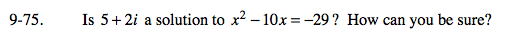Home > A2C > Chapter 9 > Lesson 9.2.1 > Problem9-75

9-75.

Is 5 + 2i a solution to x2 − 10x = −29? How can you be sure? Homework Help ✎Substitute it into the equation.

(5 + 2i)2 − 10(5 + 2i) = −29